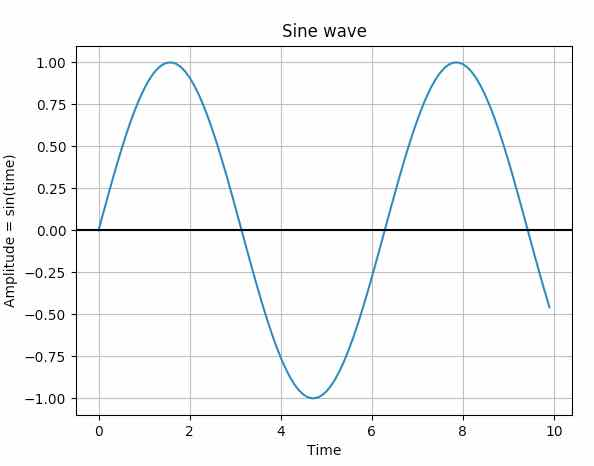# Plotting A Sine Wave Using Matplotlib And Numpy

## Overview

• Sine waves represent periodic oscillations.

• Sine waves have the shape of sine curve.

• The X-axis of the sine curve represents the time.

• The Y-axis of the sine curve represents the amplitude of the sine wave.

• The amplitude of the sine wave at any point in Y is proportional to the sine of a variable.

• The sine wave is given by the equation

A sin(ωt)

A                        - Amplitude

t                         - Time

ω (Omega)         - Frequency

• The sine curve goes through origin.

• A cycle of sine wave is complete when the position of the sine wave starts from a position and comes to the same position after attaining its maximum and minimum amplitude during its course.

• The time taken to complete one cycle is called the period of the sine wave.

• The frequency of the sine wave is given by number of cycles per second.

• ‘A’ denotes amplitude of a sine wave.

• The distance covered by a cycle measures the wavelength of the sine wave.

• The wavelength of the sine wave is denoted by λ.

• Examples of sine waves include the oscillations produced by the suspended weight on spring and the alternating current.

• NumPy has the sin() function, which takes an array of values and provides the sine value for them.

• Using the numpy sin() function and the matplotlib plot()a sine wave can be drawn.

## Example:

 import numpy as np import matplotlib.pyplot as plot   # Get x values of the sine wave time        = np.arange(0, 10, 0.1);   # Amplitude of the sine wave is sine of a variable like time amplitude   = np.sin(time)   # Plot a sine wave using time and amplitude obtained for the sine wave plot.plot(time, amplitude)   # Give a title for the sine wave plot plot.title('Sine wave')   # Give x axis label for the sine wave plot plot.xlabel('Time')   # Give y axis label for the sine wave plot plot.ylabel('Amplitude = sin(time)')   plot.grid(True, which='both')   plot.axhline(y=0, color='k')   plot.show()   # Display the sine wave plot.show()

## Output: